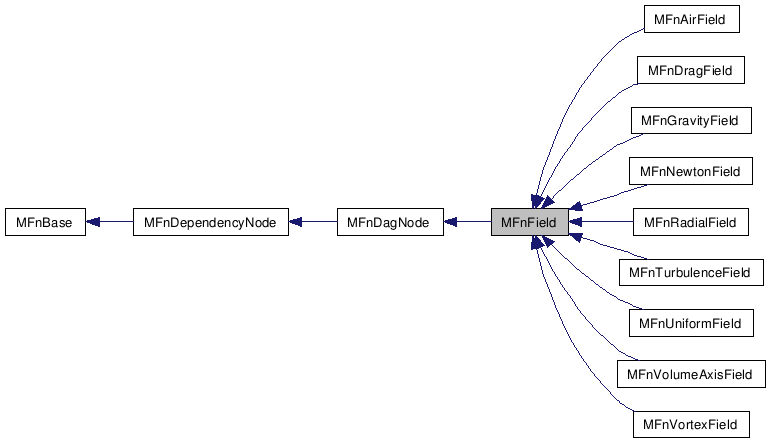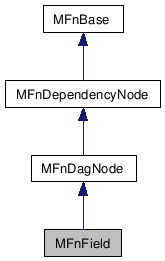# MFnField Class Reference [OpenMayaFX - API module for effects]

`#include <MFnField.h>`

Inheritance diagram for MFnField:[legend]
Collaboration diagram for MFnField:[legend]

## Detailed Description

Function set for Dynamic Fields.

Function set for creation, edit, and query of Dynamic Fields.

There are several types of dynamic fields: Air, Drag, Gravity, Newton Radial, Turbulence, Uniform, and Vortex.

## Public Member Functions

virtual MFn::Type type () const
Function set type.
virtual ~MFnField ()
Destructor.
MFnField ()
Default constructor.
MFnField (MObject &object, MStatus *ReturnStatus=NULL)
Constructor.
MFnField (const MDagPath &object, MStatus *ret=NULL)
Constructor.
MStatus getForceAtPoint (const MPointArray &point, const MVectorArray &velocity, const MDoubleArray &mass, MVectorArray &force, double deltaTime=1.0/24.0)
MStatus getForceAtPoint (const MVectorArray &point, const MVectorArray &velocity, const MDoubleArray &mass, MVectorArray &force, double deltaTime=1.0/24.0)
double magnitude (MStatus *ReturnStatus=NULL) const
MStatus setMagnitude (double mag)
double attenuation (MStatus *ReturnStatus=NULL) const
MStatus setAttenuation (double atten)
double maxDistance (MStatus *ReturnStatus=NULL) const
MStatus setMaxDistance (double maxDist)
bool perVertex (MStatus *ReturnStatus=NULL) const
MStatus setPerVertex (bool enable)
bool useMaxDistance (MStatus *ReturnStatus=NULL) const
MStatus setUseMaxDistance (bool enable)
double falloffCurve (const double param, MStatus *ReturnStatus=NULL)
bool isFalloffCurveConstantOne (MStatus *ReturnStatus=NULL)
MFnField (const MObject &object, MStatus *ret=NULL)
Constructor.

## Protected Member Functions

virtual const char * className () const
Class name.

## Constructor & Destructor Documentation

 MFnField::~MFnField ( ) ` [virtual]`

Destructor.

Class destructor.

 MFnField::MFnField ( MObject & object, MStatus * ReturnStatus = `NULL` )

Constructor.

Class constructor that initializes the function set to the given MObject.

Parameters:
 [in] object The MObject to attach the function set to [out] ReturnStatus the return status
Status Codes:

 MFnField::MFnField ( const MDagPath & object, MStatus * ReturnStatus = `NULL` )

Constructor.

Class constructor that initializes the function set to the given constant MDagPath object.

Parameters:
 [in] object The const MDagPath to attach the function set to [out] ReturnStatus The return status
Status Codes:

 MFnField::MFnField ( const MObject & object, MStatus * ReturnStatus = `NULL` )

Constructor.

Class constructor that initializes the function set to the given MObject.

Parameters:
 [in] object The MObject to attach the function set to [out] ReturnStatus the return status
Status Codes:

## Member Function Documentation

 MFn::Type MFnField::type ( ) const` [virtual]`

Function set type.

Return the class type : MFn::kField

Reimplemented from MFnDagNode.

 MStatus MFnField::getForceAtPoint ( const MPointArray & point, const MVectorArray & velocity, const MDoubleArray & mass, MVectorArray & force, double deltaTime = `1.0 / 24.0` )

Compute the force of a field on an array of points, given their position, velocity, and mass. Note that only the Air and Vortex fields require a time increment to compute forces, all other fields will ignore this argument.

This method uses MPointArray to represent the positions of points. If a point instance is in a rational form or a homogenous form, you should reset it to be in the cartesian form P(x, y, z, 1).

Parameters:
 [in] point array of positions for each point. [in] velocity array of velocities for each point. If the length of the velocity array is 0, a velocity of 0.0 is assumed for all the points. Note the velocity array is a requirement for the Air and Drag fields to compute forces. [in] mass array of mass values for each point. If the length of the mass array is 0, a mass of 1.0 is assumed for all the points. [out] force output array of forces applied to each point. If the length of the force array supplied is 0, the array is automatically resized. If the contents of the force array contains data, the computed force is added to the supplied data. This can be useful to accumulate forces of multiple fields. [in] deltaTime time increment in seconds for usage with the Air and Vortex fields. Default is (1.0 / 24.0 fps).
Returns:
Status code
Status Codes:

 MStatus MFnField::getForceAtPoint ( const MVectorArray & point, const MVectorArray & velocity, const MDoubleArray & mass, MVectorArray & force, double deltaTime = `1.0 / 24.0` )

Compute the force of a field on an array of points, given their position, velocity, and mass. Note that only the Air and Vortex fields require a time increment to compute forces, all other fields will igonore this argument.

This method uses MVectorArray to represent the positions of a point.

Parameters:
 [in] point array of positions for each point. [in] velocity array of velocities for each point. If the length of the velocity array is 0, a velocity of 0.0 is assumed for all the points. Note the velocity array is a requirement for the Air and Drag fields to compute forces. [in] mass array of mass values for each point. If the length of the mass array is 0, a mass of 1.0 is assumed for all the points. [out] force output array of forces applied to each point. If the length of the force array supplied is 0, the array is automatically resized. If the contents of the force array contains data, the computed force is added to the supplied data. This can be useful to accumulate forces of multiple fields. [in] deltaTime time increment in seconds for usage with the Air and Vortex fields. Default is (1.0 / 24.0 fps).
Returns:
Status code
Status Codes:

 double MFnField::magnitude ( MStatus * ReturnStatus = `NULL` ) const

Returns the strength of the field.

Parameters:
 [out] ReturnStatus Status code
Returns:
A value representing the strength of the field.
Status Codes:

 MStatus MFnField::setMagnitude ( double mag )

Sets the strength of the field.

Parameters:
 [in] mag A value representing the strength of the field.
Returns:
Status code
Status Codes:

 double MFnField::attenuation ( MStatus * ReturnStatus = `NULL` ) const

Returns the rate of change where the strength of the field changes as the distance between the field and the affected object increases.

Parameters:
 [out] ReturnStatus Status code
Returns:
• A positive value representing the exponent for rate of change.
• A value of 0 and the force remains constant over distance.
Status Codes:

 MStatus MFnField::setAttenuation ( double atten )

Sets the rate of change where the strength of the field changes as the distance between the field and the affected object increases.

Parameters:
 [in] atten A positive value representing the exponent for rate of change. A value of 0 and the force remains constant over distance.
Returns:
Status code
Status Codes:

 double MFnField::maxDistance ( MStatus * ReturnStatus = `NULL` ) const

Returns the maximum distance from the field at which the force of the field is exerted. The Use Max Distance setting must be turned on for maximum distance to take effect. The maximum distance is scaled by the falloff curve's non-zero range.

Parameters:
 [out] ReturnStatus Status code
Returns:
A value representing distance in internal linear units.
Status Codes:

 MStatus MFnField::setMaxDistance ( double dist )

Sets the maximum distance from the field at which the force of the field is exerted. The Use Max Distance setting must be turned on for maximum distance to take effect.

Parameters:
 [in] dist A value representing distance in internal linear units.
Returns:
Status code
Status Codes:

 bool MFnField::perVertex ( MStatus * ReturnStatus = `NULL` ) const

Returns true if the field exerts its force on each individual point (cv, particle, vertex) equally. Returns false if the force is exerted only from the geometric center of the object or set of points.

Parameters:
 [out] ReturnStatus Status code
Returns:
• true Force is applied to each individual point.
• false Force is applied from the geometric center.
Status Codes:

 MStatus MFnField::setPerVertex ( bool enable )

Enables the field to exert its force on each individual point (cv, particle, vertex) equally. Otherwise, the force is exerted only from the geometric center of the object or set of points.

Parameters:
 [in] enable Flag to enable or disable individual point forces.
Returns:
Status code
Status Codes:

 bool MFnField::useMaxDistance ( MStatus * ReturnStatus = `NULL` ) const

Returns true if the field will use the maximum distance setting to determine the area of influence.

Parameters:
 [out] ReturnStatus Status code
Returns:
• true Field uses the maximum distance setting.
• false Field ignores the maximum distance setting.
Status Codes:

 MStatus MFnField::setUseMaxDistance ( bool enable )

Enables the field to use the maximum distance setting to determine the area of influence.

Parameters:
 [in] enable Flag to enable or disable maximum distance.
Returns:
Status code
Status Codes:

 double MFnField::falloffCurve ( const double param, MStatus * ReturnStatus = `NULL` )

Returns falloff given the param in [0,1]. This is enabled if the use the maximum distance is enabled.

Parameters:
 [in] param Parameter [out] ReturnStatus Status code
Returns:
The falloff value given the param.
Status Codes:

 bool MFnField::isFalloffCurveConstantOne ( MStatus * ReturnStatus = `NULL` )

Returns true if falloffCurve is a constant one (default) or false if not.

Parameters:
 [out] ReturnStatus Status code
Returns:
If falloff curve always returns a constant one
Status Codes:

 Autodesk® Maya® 2009 © 1997-2008 Autodesk, Inc. All rights reserved. Generated with1.5.6